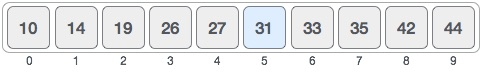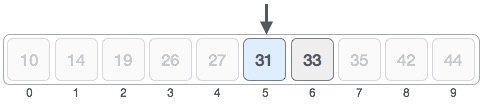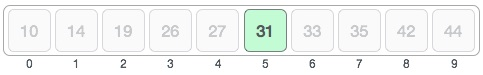## Binary Search - Data Structures and Algorithms

Binary search is a fast search algorithm with run-time complexity of Ο(log n). This search algorithm works on the principle of divide and conquer. For this algorithm to work properly, the data collection should be in the sorted form.

Binary search looks for a particular item by comparing the middle most item of the collection. If a match occurs, then the index of item is returned. If the middle item is greater than the item, then the item is searched in the sub-array to the left of the middle item. Otherwise, the item is searched for in the sub-array to the right of the middle item. This process continues on the sub-array as well until the size of the subarray reduces to zero.

## 1. How Binary Search Works?

For a binary search to work, it is mandatory for the target array to be sorted. We shall learn the process of binary search with a pictorial example. The following is our sorted array and let us assume that we need to search the location of value 31 using binary search.First, we shall determine half of the array by using this formula:

mid = low + (high - low) / 2

Here it is, 0 + (9 - 0 ) / 2 = 4 (integer value of 4.5). So, 4 is the mid of the array.Now we compare the value stored at location 4, with the value being searched, i.e. 31. We find that the value at location 4 is 27, which is not a match. As the value is greater than 27 and we have a sorted array, so we also know that the target value must be in the upper portion of the array.We change our low to mid + 1 and find the new mid value again.

low = mid + 1

mid = low + (high - low) / 2

Our new mid is 7 now. We compare the value stored at location 7 with our target value 31.The value stored at location 7 is not a match, rather it is more than what we are looking for. So, the value must be in the lower part from this location.Hence, we calculate the mid again. This time it is 5.We compare the value stored at location 5 with our target value. We find that it is a match.We conclude that the target value 31 is stored at location 5.

Binary search halves the searchable items and thus reduces the count of comparisons to be made to very less numbers.

## 2. Pseudocode

The pseudocode of binary search algorithms should look like this :

Procedure binary_search

A ← sorted array

n ← size of array

x ← value to be searched

Set lowerBound = 1

Set upperBound = n

if upperBound < lowerBound

EXIT: x does not exists.

set midPoint = lowerBound + ( upperBound - lowerBound ) / 2

if A[midPoint] < x

set lowerBound = midPoint + 1

if A[midPoint] > x

set upperBound = midPoint - 1

if A[midPoint] = x

EXIT: x found at location midPoint

end while

end procedure

New
Related